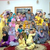0
Ülevaatamisel

# Input string was not in a correct format

panbac88 6 aastat tagasi Bugs uuendatud 6 aastat tagasi
Hi,

I am using OxyPlot.WIndowsUniversal 2014.1.546.0 in a win8.1 project. I am trying to create a chart witth a category axis, a linear axis and a line series. However in the drawing space of the chart I am getting the following error.
```OxyPlot exception: Input string was not in a correct format.
Stack trace:
An exception of tpy[e System.FormatException was thrown when updating the plot model.
at System.Number.ParseDouble(String value, NumberStyles options, NumberFormatInfo numfmt)
at System.String.System.IConvertible.ToDouble(IFormatProvider provider)
at System.Convert.ToDouble(Object value)
at OxyPlot.Axes.Axis.ToDouble(Object value)
at OxyPlot.ReflectionExtensions.AddRange(List'1 target, IEnumerable itemsSource, String dataFieldX, String dataFieldY)
at OxyPlot.Series.DataPointSeries.UpdateItemsSourcePoints()
at OxyPlot.Series.DataPointSeries.UpdateData()
at OxyPlot.PlotModel.OxyPlot.IPlotModel.Update(Boolean updateData)
```
My code is this:
```var chartModel = new PlotModel();
CategoryAxis categAxis = new CategoryAxis(); categAxis.Position = AxisPosition.Bottom;
categAxis.ItemsSource = chartvm.DateValues;
categAxis.LabelField = "MN";
LinearAxis linAxis = new LinearAxis();
linAxis.Position = AxisPosition.Left;
var series = new LineSeries
{
Color = OxyColor.FromArgb(255, 78, 154, 6),
DataFieldX = "MN",
DataFieldY = "Value",
ItemsSource = chartvm.DateValues
};
plotView.Model = chartModel;
DateValues is an ObservableCollection of type DateValue and DateValue is defined below.
public DateValue(DateTime dateTime, double value, string monthName, bool isFiller) {
_Date = dateTime;
_Value = value;
_mn = monthName;
_isFiller = isFiller;
}
private DateTime _Date;
public DateTime Date
{
get { return _Date; }
set { _Date = value; }
}
private double _Value;
public double Value
{
get { return _Value; }
set { _Value = value; }
}
private string _mn;
public string MN
{
get { return _mn; }
set { _mn = value; }
}
private bool _isFiller;
public bool IsFiller
{
get { return _isFiller; }
set { _isFiller = value; }
}
```

I can't understand why i'm getting the error. I have verified that the data is correct since it is displayed in a list next to the chart.

Thanks in advance for any help. It will be really appreciated.Ülevaatamisel
What is the value of the object to be parsed (the argument of the ToDouble) method. To me the code looks OK, and it should always be possible to convert double to double...I am not calling the ToDouble method myself. It is called internally. Is it posible that the string value DateValue.MN is being parsed as a double?? That is the only thing I can think of that makes sense.

Can anybody reproduce the problem?DataFieldX = "MN",
DataFieldY = "Value",

to

DataFieldX = "Value",
DataFieldY = "MN",

and removing
categAxis.ItemsSource = chartvm.DateValues;
categAxis.LabelField = "MN";

The same error occued again.I also have same problem when using LineSeries with CategoryAxis and LinearAxis.
It seem that when using LineSeries, X & Y axis must be in double.
My solution is to add dummy field in double into the model
Example
Class DateValue {
double Value = 10.5, 30.7,10.3
string MonthName = "Jan", "Feb", "Mar"